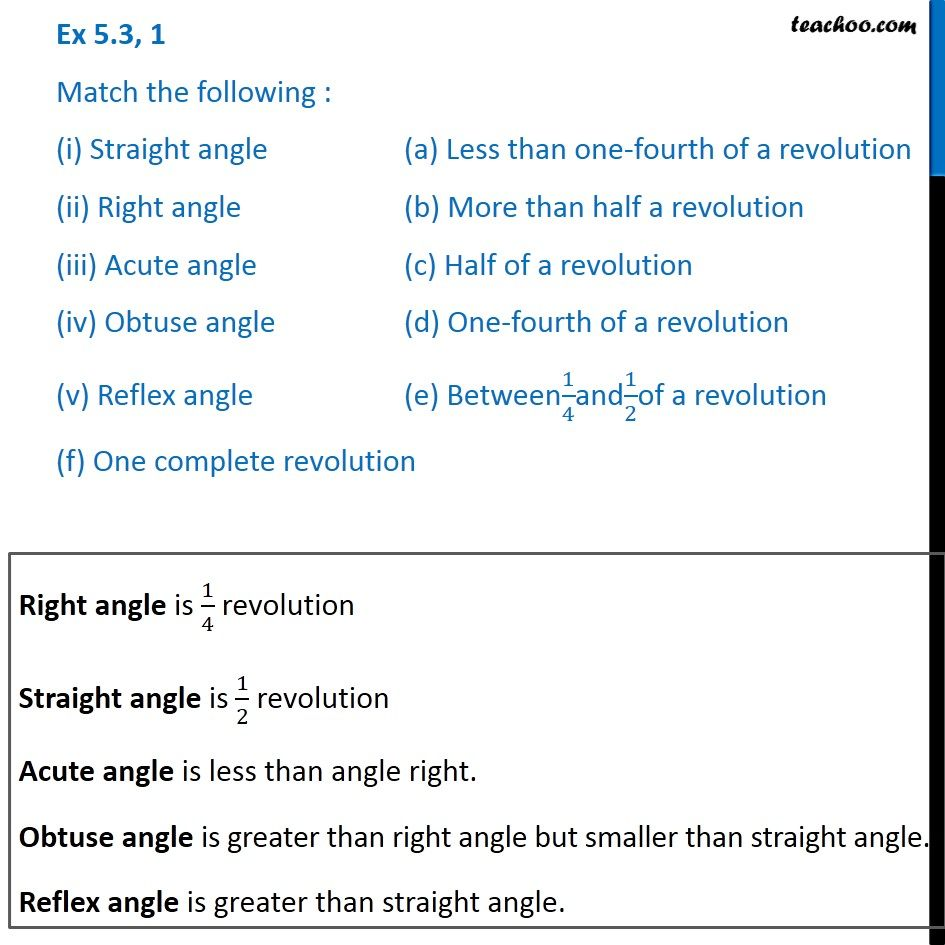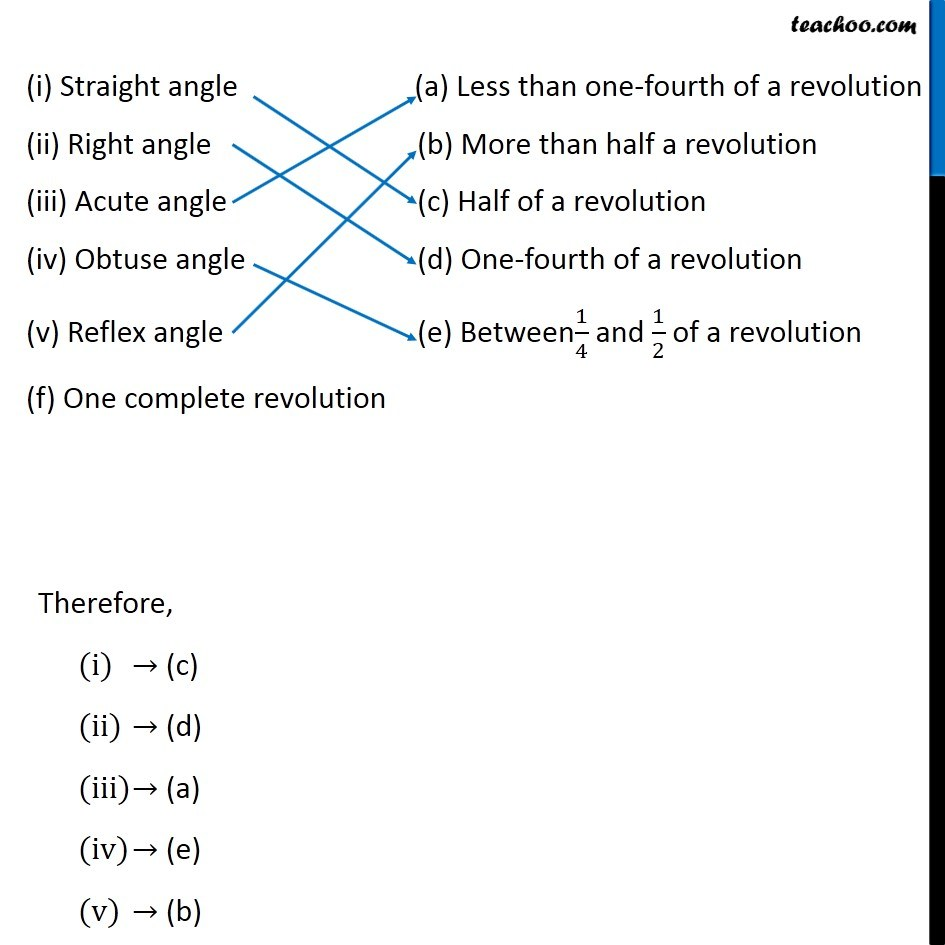Ex 5.3

Chapter 5 Class 6 Understanding Elementary Shapes
Serial order wiseLearn in your speed, with individual attention - Teachoo Maths 1-on-1 Class

### Transcript

Ex 5.3, 1 Match the following : (i) Straight angle (a) Less than one-fourth of a revolution (ii) Right angle (b) More than half a revolution (iii) Acute angle (c) Half of a revolution (iv) Obtuse angle (d) One-fourth of a revolution (v) Reflex angle (e) Between1/4and1/2of a revolution (f) One complete revolution Right angle is 1/4 revolution Straight angle is 1/2 revolution Acute angle is less than angle right. Obtuse angle is greater than right angle but smaller than straight angle. Reflex angle is greater than straight angle. (i) Straight angle (a) Less than one-fourth of a revolution (ii) Right angle (b) More than half a revolution (iii) Acute angle (c) Half of a revolution (iv) Obtuse angle (d) One-fourth of a revolution (v) Reflex angle (e) Between1/4 and 1/2 of a revolution (f) One complete revolution Therefore, (c) (d) (a) (e) (b)